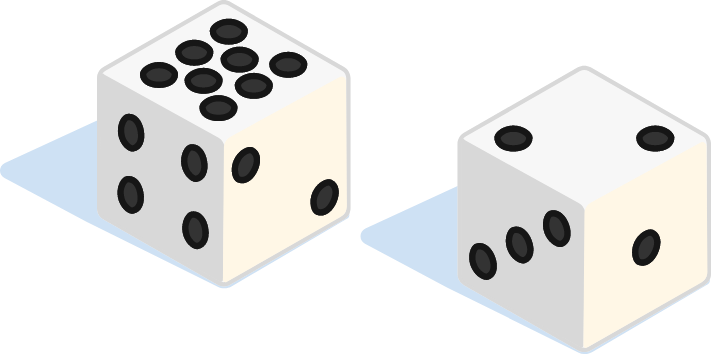# Christmas Streak 10/88: Two Strange To Be Strange

Probability Level 3

I have two distinct, fair, six-sided dice that have positive integers on their sides. Neither of the dice is a "normal" die (i.e., with 1, 2, 3, 4, 5, and 6 on the sides).

Interestingly, the probability distribution of the sum of rolling these two dice is exactly the same as that of the sum of rolling 2 "normal" dice.

Let the numbers on the first die be $(a_1, a_2, a_3, a_4, a_5, a_6),$ and those on the second $(b_1, b_2, b_3, b_4, b_5, b_6),$ where $a_1\leq a_2 \leq\cdots\leq a_6$ and $b_1\leq b_2 \leq \cdots\leq b_6.$

Submit your answer as the product of these two 6-digit integers: $\overline{a_1 a_2 a_3 a_4 a_5 a_6} \times \overline{b_1 b_2 b_3 b_4 b_5 b_6 }.$The dice above have 1,1,2,2,4,8 and 1,2,3,3,4,4 on their sides. Their sum can produce the same numbers (2-12) as the sum of two ordinary dice, but not with the same probability distribution.

×

Problem Loading...

Note Loading...

Set Loading...